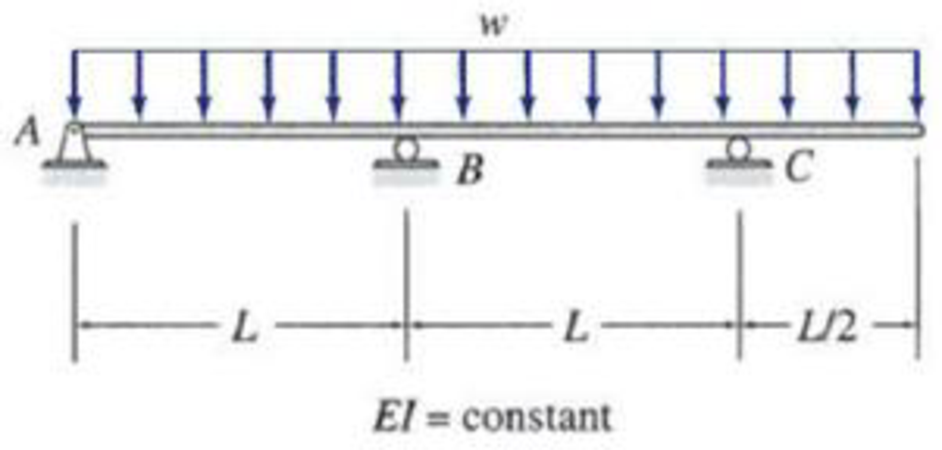# 13.37 through 13.45 Determine the reactions and draw the shear and bending moment diagrams for the structures shown in Figs. P13.37–P13.45 using the method of consistent deformations. FIG. P13.17

#### Solutions

Chapter
Section
Chapter 13, Problem 17P
Textbook Problem
81 views

## 13.37 through 13.45 Determine the reactions and draw the shear and bending moment diagrams for the structures shown in Figs. P13.37–P13.45 using the method of consistent deformations.FIG. P13.17

To determine

Calculate the support reactions for the given structure using method of consistent deformation.

Sketch the shear and bending moment diagrams for the given structure.

### Explanation of Solution

Given information:

The structure is given in the Figure.

Apply the sign conventions for calculating reactions, forces and moments using the three equations of equilibrium as shown below.

• For summation of forces along x-direction is equal to zero (Fx=0), consider the forces acting towards right side as positive (+) and the forces acting towards left side as negative ().
• For summation of forces along y-direction is equal to zero (Fy=0), consider the upward force as positive (+) and the downward force as negative ().
• For summation of moment about a point is equal to zero (Matapoint=0), consider the clockwise moment as positive when moment taken from left and the counter clockwise moment as positive when moment taken from right.

Calculation:

The beam and the loading are symmetric since only analyze only the right half DG of the beam.

Sketch the free body diagram of the right half DG of the beam as shown in Figure 1.

Refer Figure 1.

Find the degree of indeterminacy of the structure:

Degree of determinacy of the beam is equal to the number of unknown reactions minus the number of equilibrium equations.

The beam is supported by 4 support reactions and the number of equilibrium equations is 3.

Therefore, the degree of indeterminacy of the beam is i=1.

Select the vertical reaction By at the support B as redundant.

Release the support B consider the notation of moments due to external load as MO.

Sketch the free body diagram of primary beam subjected to external loading without support B as shown in Figure 2.

Refer Figure 2.

Find the reactions at the supports without considering support B using equilibrium equations:

Summation of moments of all forces about A is equal to 0.

MD=0CyO(2L)w(52L)(52L2)=02LCyO=258wL2CyO=25wL16()

Summation of forces along y-direction is equal to 0.

+Fx=0AyOw(52L)+25wL16=0AyO=15wL16()

For unit value of the unknown redundant By:

Consider the notation of moments due to external load as mB.

Sketch the free body diagram of primary beam Subjected to unit value of redundant By as shown in Figure 3.

Refer Figure 3.

Find the support reaction when unit load applied at B.

The load acts center of the both supports.

Ayu=Cyu=12()

Find the moment equation of the beam for different sections on the beam.

Consider a section XX in the portion DE of the primary structure at a distance of x from D.

Refer Figure 1.

Draw the primary structure with section XX as shown in Figure 4.

Refer Figure 4.

Portion AC.

Consider origin as A. (0x2L).

Find the moment at section XX in the portion DE as shown in Figure 5.

MO=15wL16xwx22

Tabulate the moment equation of different segment of beam as in Table 1.

 Segment x-coordinate MO mBY Origin Limits AB A 0−L 15wL16x−wx22 −x2 BC B L−2L 15wL16x−wx22 −x2+1(x−L) CD D 0−L2 wx22 0

The vertical deflection at point B due to external loading is ΔBO and the flexibility coefficient representing the deflection at B due to unit value of redundant By is fBB.

Calculate the value of ΔBO using the equation as follows:

ΔBO=ΣMOmYEIdx=1EI{0L(15wL16xwx22)(x2)dx+L2L(15wL16xwx22)(x2+1(xL))dx}=1EI{0L(15wLx232+wx34)dx+L2L(15wL16xwx22)(x2L)dx}=1EI{0L(15wLx232+wx34)dx+L2L(15wLx232wx3415wL216x+wLx22)dx}

ΔBO=1EI[(15wLx396+wx416)0L+(15wLx396wx41615wL2x232+wLx36)L2L]=1EI[(15wL496+wL416)+(120wL49616wL41660wL432+8wL46)(15wL496wL41615wL432+wL46)]=1EI[15wL496+wL416+120wL49616wL41660wL432+8wL4615wL496+wL416+15wL432wL46]=1EI(17wL496)=17wL496EI

Calculate the value of fBB using the equation as follows:

f

### Still sussing out bartleby?

Check out a sample textbook solution.

See a sample solution

#### The Solution to Your Study Problems

Bartleby provides explanations to thousands of textbook problems written by our experts, many with advanced degrees!

Get Started

Find more solutions based on key concepts
What do we mean by a uniform series payment?

Engineering Fundamentals: An Introduction to Engineering (MindTap Course List)

What e user applications? Suggest three examples that could boost user productivity.

Systems Analysis and Design (Shelly Cashman Series) (MindTap Course List)

In addition to keeping the antivirus programs virus definitions current, what other ways can you protect a comp...

Enhanced Discovering Computers 2017 (Shelly Cashman Series) (MindTap Course List)

Convert P = 5.00 atm into Pa, bar, and psia.

Fundamentals of Chemical Engineering Thermodynamics (MindTap Course List)

What is in-memory database processing, and what advantages does it provide?

Principles of Information Systems (MindTap Course List)

When would a radial-arm drill press most likely be used?

Precision Machining Technology (MindTap Course List)

What two welding processes are semiautomatic processes?

Welding: Principles and Applications (MindTap Course List)

If your motherboard supports ECC DDR3 memory, can you substitute non-ECC DDR3 memory?

A+ Guide to Hardware (Standalone Book) (MindTap Course List)